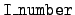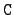Next: The format for `mcf.asc' Up: The format of the Previous: Reaction header blocks   Contents

## I_number blocks

For each reaction there several blocks of data. The section of nhead devoted to data location and identification provides the number numi of these I_number blocks and their locations. The content of one of these blocks depends on the value of I_number as follows. Again, the data locations here are in terms of the start of the data for this target.= 0:
For the reaction cross sections we have two offsets.

data_fwa:
The location of the start of the (energy, cross section) data for this reaction.

data_lwa:
The location of the end of this data.= 1:
For the angular distributions the data consists of pairs (cosine, probability density) for a number of incident energies. The corresponding set in nhead contains the following information.

data_fwa:
The location of the start of the angular distribution data for this reaction. This is the location of the incident energies.

data_lwa:
The location of the end of the angular distribution data.

max_cos_prob:
The maximum number of words of angular distribution data for each incident energy.

num_engy_in:
The number of incident energies.

I1_data_fwa:
Here are num_engy_in numbers: the location of the start of the angular distribution data for each incident energy.= 3:
This block of data corresponds to correlated probability of angle and energy of the secondary particle. The data block starts with the incident energies. For each incident energy we then have the cosines, followed by the corresponding pairs (energy of secondary particle, probability density). This set in nhead contains the following information.

data_fwa:
The location of the start of the correlated distribution data for this reaction. This is the location of the incident energies.

data_lwa:
The location of the end of the correlated distribution data.

num_engy_in:
The number of incident energies.

n3cosmax:
The maximum number of cosines for each incident energy.

nE_out_max:
The maximum amount of (E', probability density) data for each incident energy and each cosine. There now follows num_engy_in blocks.

I3_ptr:
The location of the cosines for this incident energy.

n3cos:
The number of cosines for this incident energy.

e_prob_ptr:
We have here n3cos locations of the starts of the energy distribution data for this incident energy and cosine.= 4:
For the energy distributions the data consists of pairs (energy of secondary particle, probability density) for a number of incident energies. The corresponding set in nhead contains the following information.

data_fwa:
The location of the start of the energy distribution data for this reaction.

data_lwa:
The location of the end of the energy distribution data.

lmax:
The order of the Legendre expansion of the flux. This must be zero.

max_num_engy_in:
Unused by mcfgen.

lep:
The maximum number of words of energy distribution data for each incident energy.

energy_fwa:
The location of the incident energies.

num_engy_in:
The number of incident energies.

I4_data_fwa:
A collection of num_engy_in locations of the starts of the energy distribution data for this incident energy.= 7:
The data is pairs (energy, number of neutrons per fission), prompt or delayed, and we have two offsets.

data_fwa:
The location of the start of the neutron multiplicities.

data_lwa:
The location of the end of the neutron multiplicity data.= 9:
The data is (energy, number of photons emitted), and we have two offsets.

data_fwa:
The location of the start of the multiplicity data for this reaction.

data_lwa:
The location of the end of the multiplicity data.= 10:
For the pairs (incident energy, energy deposition to secondary particle) we have two offsets.

data_fwa:
The location of the start of the energy deposition data for this reaction.

data_lwa:
The location of the end of the energy deposition data.= 11:
For the pairs (incident energy, energy deposition to the residual nucleus) we have two offsets. This data is currently not used.

data_fwa:
The location of the start of the energy deposition data for this reaction.

data_lwa:
The location of the end of the energy deposition data.= 12:
For the energy production by the non-elastic reactions the data comes in pairs (incident energy, energy production), and we have two offsets. This data is used only with production cross sections (= 5).

data_fwa:
The location of the start of the data for this reaction.

data_lwa:
The location of the end of this data.= 941:
For the coherent scattering form factors the data is (energy, form factor), and we have two offsets.

data_fwa:
The location of the start of the form factor data for this reaction.

data_lwa:
The location of the end of the form factor data.= 942:
For the incoherent scattering form factors the information is that same as for= 941.Next: The format for `mcf.asc' Up: The format of the Previous: Reaction header blocks   Contents